Next: Hemispherical Ocean Tides Up: Terrestrial Ocean Tides Previous: Gyroscopic Coefficients

# Proudman Equations

We can automatically satisfy the boundary condition (12.209) by writing(12.281)

Likewise, we can automatically satisfy the boundary condition (12.210) by writing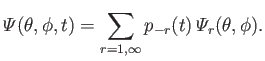(12.282)

It follows from Equations (12.211), (12.212), (12.227), and (12.235) that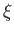(12.283)(12.284)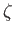(12.285)

Let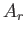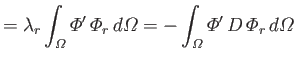(12.286)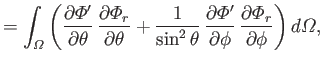(12.287)

where use has been made of Equations (12.235) and (12.236). It follows from Equations (12.217) and (12.218) that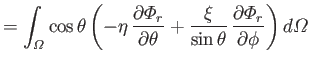(12.288)

However, the second term on the right-hand side of the previous equation integrates to zero with the aid of Equation (12.216). Hence, we are left with(12.289)

Finally, Equations (12.276), (12.282), (12.288), and (12.289) can be combined with the previous equation to give(12.290)

Let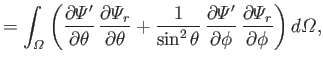(12.291)

where use has been made of Equations  (12.216) and (12.248). It follows from Equations (12.217) and (12.218) that(12.292)

However, the second term on the right-hand side of the previous equation integrates to zero with the aid of Equation (12.249). Hence, we are left with(12.293)

Finally, Equations (12.279), (12.285), (12.288), and (12.289) can be combined with the previous equation to give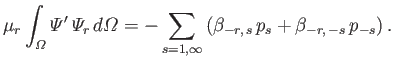(12.294)

Operating on Equations (12.228) and (12.229) with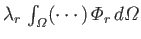and with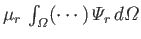, respectively, yields the so-called Proudman equations (Proudman 1916):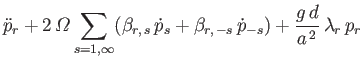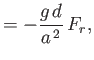(12.295)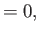(12.296)

where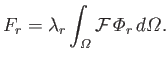(12.297)

Here, use has been made of Equations (12.240), (12.244), (12.250), (12.286), (12.287), (12.290), (12.295), and (12.299). Finally, it follows from Equations (12.138), (12.189), and (12.290) that(12.298)

where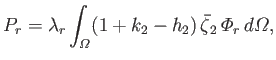(12.299)

and(12.300)

with(12.301)

Here,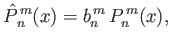(12.302)

where(12.303)

It follows from Equation (12.136) that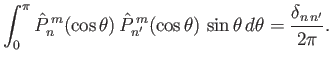(12.304)

Consider the response of the ocean to a particular harmonic of the tide generating potential for which(12.305)

Assuming a commontime dependence of the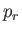and the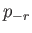, Equations (12.300) and (12.301) yieldand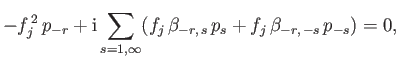(12.306)

whereand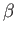are defined in Equations (12.173) and (12.174), respectively.

The task in hand (see Section 12.13) is to solve the Laplace tidal equations, (12.186)-(12.189), subject to the constraint (12.190). Our basic approach is to expand the fields appearing in the Laplace tidal equations--namely,,, and--in terms of a set of basis eigenfunctions--the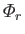and the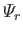(see Section 12.17)--that are defined in such a manner as to automatically satisfy the boundary conditions. The expansion is specified in Equations (12.288)-(12.290). The Laplace tidal equations then reduce to the Proudman equations, (12.300)-(12.301), which are a set of coupled ordinary differential equations for the weights in the expansion--theand the. For a particular harmonic of the tide generating potential, the weights all oscillate at the same frequency, and the Proudman equations reduce further to give two coupled matrix equations for the amplitudes of the weights--see Equations (12.311) and (12.312). In order to solve the matrix equations, we need to calculate the gyroscopic coefficients,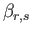,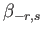,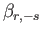,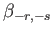, as well as the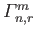. These quantities are integrals involving the eigenfunctions and the associated Legendre functions, and are defined in Equations (12.276), (12.279), (12.282), (12.285), and (12.306), respectively.Next: Hemispherical Ocean Tides Up: Terrestrial Ocean Tides Previous: Gyroscopic Coefficients
Richard Fitzpatrick 2016-03-31